# i have problem in gas sensor

• hi guys.
i use gas sketch in site and several change this.but i have problem. after times sensor can not show any change . first (after upload code) this is true . but after some days. i test this with gas. but this is fix 0 and dont any change .
finally i use this sketch for send message every 60 s . in domoticz controller i have message every 60 s but number of ppm dont change and this is 0 always.
i test with some sensors and some arduinos but dont resolve this problem. so i think this problem is related to Calculation of sensors.
also when i put sensor in place of gas , LED in gas sensor go to on ! so sensors is true , also every 60s i see a report in domoticz log....
this is last sketch :

``````#define MY_DEBUG
#define MY_RF24_CHANNEL 0
#define MY_NODE_ID 5
#define MY_REPEATER_FEATURE

#include <MySensors.h>
#include <avr/wdt.h>

unsigned long time_m;
unsigned long a , b , c;

#define     CHILD_ID_MQ                   0
/************************Hardware Related Macros************************************/
#define     MQ_SENSOR_ANALOG_PIN         (0)  //define which analog input channel you are going to use
#define         RL_VALUE                     (5)     //define the load resistance on the board, in kilo ohms
#define         RO_CLEAN_AIR_FACTOR          (9.83)  //RO_CLEAR_AIR_FACTOR=(Sensor resistance in clean air)/RO,
//which is derived from the chart in datasheet
/***********************Software Related Macros************************************/
#define         CALIBARAION_SAMPLE_TIMES     (50)    //define how many samples you are going to take in the calibration phase
#define         CALIBRATION_SAMPLE_INTERVAL  (500)   //define the time interal(in milisecond) between each samples in the
//cablibration phase
#define         READ_SAMPLE_INTERVAL         (50)    //define how many samples you are going to take in normal operation
#define         READ_SAMPLE_TIMES            (5)     //define the time interal(in milisecond) between each samples in
//normal operation
/**********************Application Related Macros**********************************/
#define         GAS_LPG                      (0)
#define         GAS_CO                       (1)
#define         GAS_SMOKE                    (2)
/*****************************Globals***********************************************/
//unsigned long SLEEP_TIME = 30000; // Sleep time between reads (in milliseconds)
//VARIABLES
float Ro = 10000.0;    // this has to be tuned 10K Ohm
int val = 0;           // variable to store the value coming from the sensor
float valMQ = 0.0;
float lastMQ = 0.0;
float           LPGCurve  =  {2.3, 0.21, -0.47}; //two points are taken from the curve.
//with these two points, a line is formed which is "approximately equivalent"
//to the original curve.
//data format:{ x, y, slope}; point1: (lg200, 0.21), point2: (lg10000, -0.59)
float           COCurve  =  {2.3, 0.72, -0.34};  //two points are taken from the curve.
//with these two points, a line is formed which is "approximately equivalent"
//to the original curve.
//data format:{ x, y, slope}; point1: (lg200, 0.72), point2: (lg10000,  0.15)
float           SmokeCurve = {2.3, 0.53, -0.44}; //two points are taken from the curve.
//with these two points, a line is formed which is "approximately equivalent"
//to the original curve.
//data format:{ x, y, slope}; point1: (lg200, 0.53), point2:(lg10000,-0.22)

MyMessage msg(CHILD_ID_MQ, V_LEVEL);

void setup()
{
Ro = MQCalibration(MQ_SENSOR_ANALOG_PIN);         //Calibrating the sensor. Please make sure the sensor is in clean air
wdt_enable(WDTO_4S);
}

void presentation() {
// Send the sketch version information to the gateway and Controller
sendSketchInfo("Gas", "1.0");

// Register all sensors to gateway (they will be created as child devices)
present(CHILD_ID_MQ, S_AIR_QUALITY);
}

void loop()
{
time_m = millis();
a = time_m / 1000;
b = a % 40;
c = a % 60;
if (b == 0) {
uint16_t valMQ = MQGetGasPercentage(MQRead(MQ_SENSOR_ANALOG_PIN) / Ro, GAS_CO);
Serial.println(val);

Serial.print("LPG:");
Serial.print( "ppm" );
Serial.print("    ");
Serial.print("CO:");
Serial.print( "ppm" );
Serial.print("    ");
Serial.print("SMOKE:");
Serial.print( "ppm" );
Serial.print("\n");
if (valMQ != lastMQ) {
send(msg.set((int16_t)ceil(valMQ)));
lastMQ = ceil(valMQ);

}}

if (c == 0) {
uint16_t valMQ = MQGetGasPercentage(MQRead(MQ_SENSOR_ANALOG_PIN) / Ro, GAS_CO);
send(msg.set((int16_t)ceil(valMQ)));
wait(1000);
}

wdt_reset();
// wait(SLEEP_TIME); //sleep for: sleepTime

}

/****************** MQResistanceCalculation ****************************************
Output:  the calculated sensor resistance
Remarks: The sensor and the load resistor forms a voltage divider. Given the voltage
across the load resistor and its resistance, the resistance of the sensor
could be derived.
************************************************************************************/
{
}

/***************************** MQCalibration ****************************************
Input:   mq_pin - analog channel
Output:  Ro of the sensor
Remarks: This function assumes that the sensor is in clean air. It use
MQResistanceCalculation to calculates the sensor resistance in clean air
and then divides it with RO_CLEAN_AIR_FACTOR. RO_CLEAN_AIR_FACTOR is about
10, which differs slightly between different sensors.
************************************************************************************/
float MQCalibration(int mq_pin)
{
int i;
float val = 0;

for (i = 0; i < CALIBARAION_SAMPLE_TIMES; i++) {      //take multiple samples
delay(CALIBRATION_SAMPLE_INTERVAL);
}
val = val / CALIBARAION_SAMPLE_TIMES;                 //calculate the average value

val = val / RO_CLEAN_AIR_FACTOR;                      //divided by RO_CLEAN_AIR_FACTOR yields the Ro
//according to the chart in the datasheet

return val;
}
Input:   mq_pin - analog channel
Output:  Rs of the sensor
Remarks: This function use MQResistanceCalculation to caculate the sensor resistenc (Rs).
The Rs changes as the sensor is in the different consentration of the target
gas. The sample times and the time interval between samples could be configured
by changing the definition of the macros.
************************************************************************************/
{
int i;
float rs = 0;

for (i = 0; i < READ_SAMPLE_TIMES; i++) {
}

return rs;
}

/*****************************  MQGetGasPercentage **********************************
Input:   rs_ro_ratio - Rs divided by Ro
gas_id      - target gas type
Output:  ppm of the target gas
Remarks: This function passes different curves to the MQGetPercentage function which
calculates the ppm (parts per million) of the target gas.
************************************************************************************/
int MQGetGasPercentage(float rs_ro_ratio, int gas_id)
{
if ( gas_id == GAS_LPG ) {
return MQGetPercentage(rs_ro_ratio, LPGCurve);
} else if ( gas_id == GAS_CO ) {
return MQGetPercentage(rs_ro_ratio, COCurve);
} else if ( gas_id == GAS_SMOKE ) {
return MQGetPercentage(rs_ro_ratio, SmokeCurve);
}

return 0;
}

/*****************************  MQGetPercentage **********************************
Input:   rs_ro_ratio - Rs divided by Ro
pcurve      - pointer to the curve of the target gas
Output:  ppm of the target gas
Remarks: By using the slope and a point of the line. The x(logarithmic value of ppm)
of the line could be derived if y(rs_ro_ratio) is provided. As it is a
logarithmic coordinate, power of 10 is used to convert the result to non-logarithmic
value.
************************************************************************************/
int  MQGetPercentage(float rs_ro_ratio, float *pcurve)
{
return (pow(10, ( ((log(rs_ro_ratio) - pcurve) / pcurve) + pcurve)));
}`````````

• @Reza
there are two problems with MQ-2 sensors :
2 are you sure that RL_VALUE is 5 (kOhm) in your MQ-2 board? In my is 1, the one labeled 102 in the pic.

Regards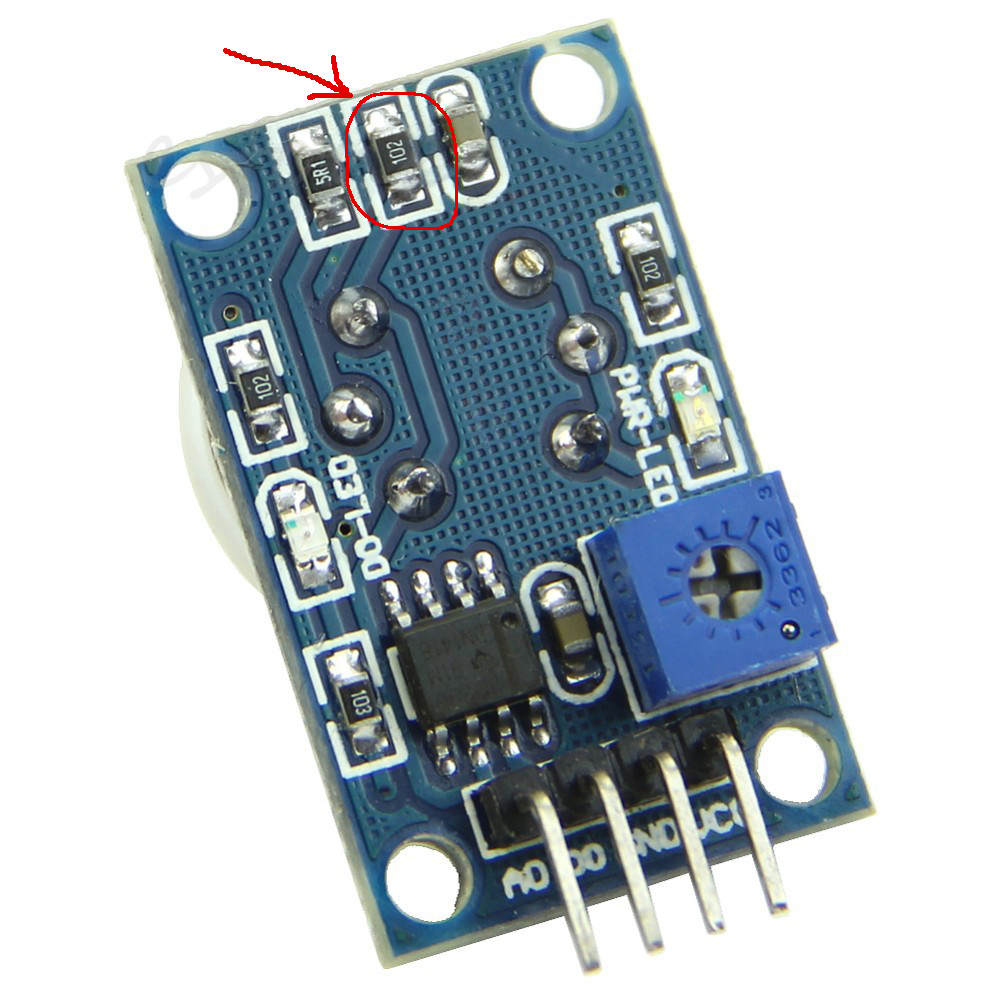• @zampedro
hi thank you for help me
about 2 , my sensor is different with you . this is my sensor (MQ2):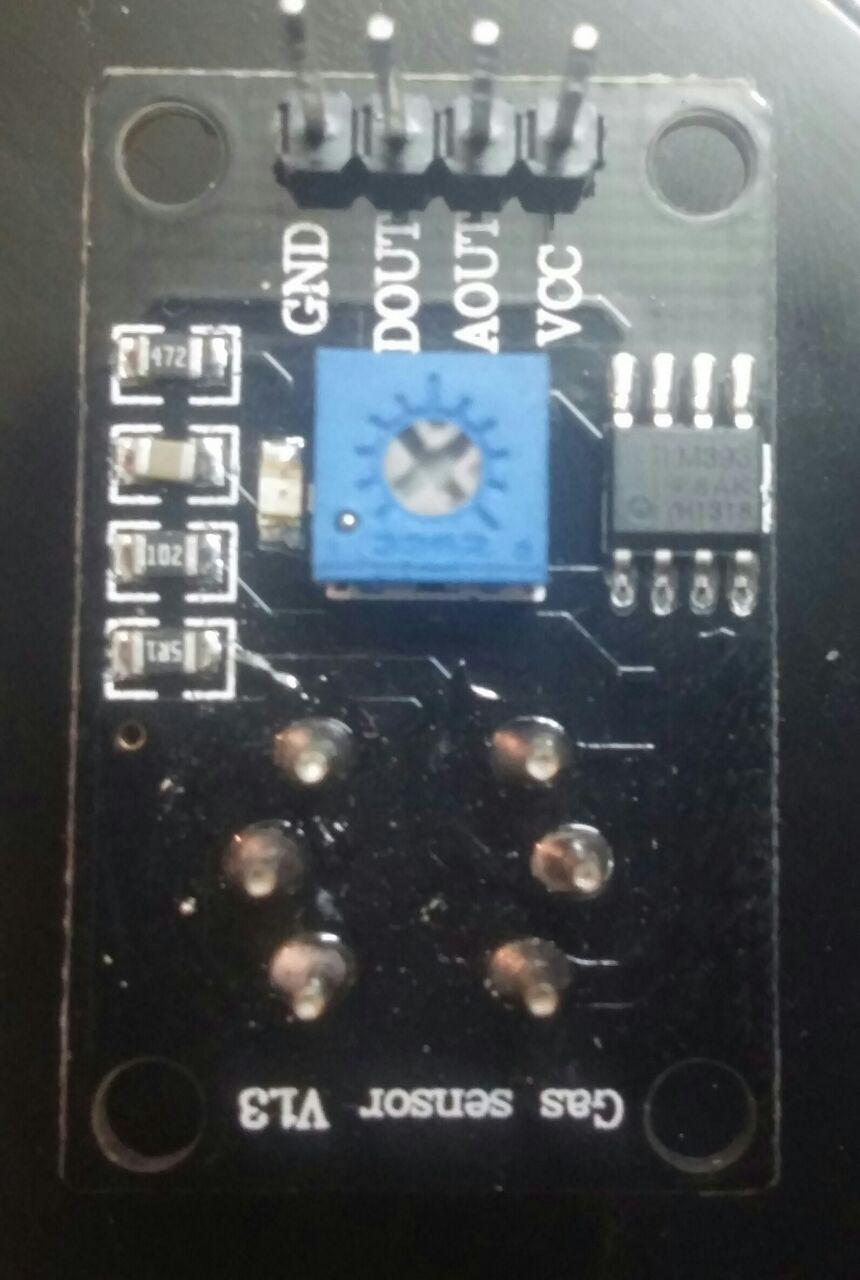• 1 look at the last line of the sketch:

``````  return (pow(10, ( ((log(rs_ro_ratio) - pcurve) / pcurve) + pcurve)));
}``
``````

change to:

``````  return (pow(10, ( ((log10(rs_ro_ratio) - pcurve) / pcurve) + pcurve)));
}``
``````

2 RL_VALUE in your MQ2 board should be 1 (kohm), R2 in the schematic.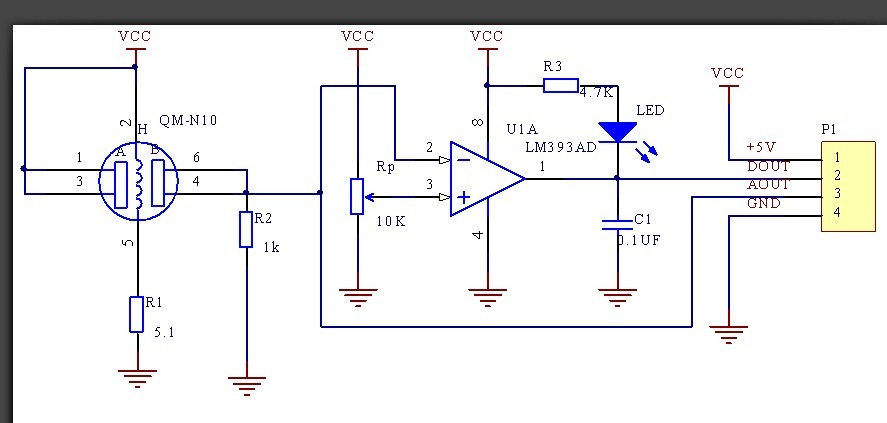• @zampedro thank you for help in 1.i change this .
but about 2. that is my sensor pic.is that my R2 in board 1K ? i am weak in electronic.if this is not so what am i do ? thank you for help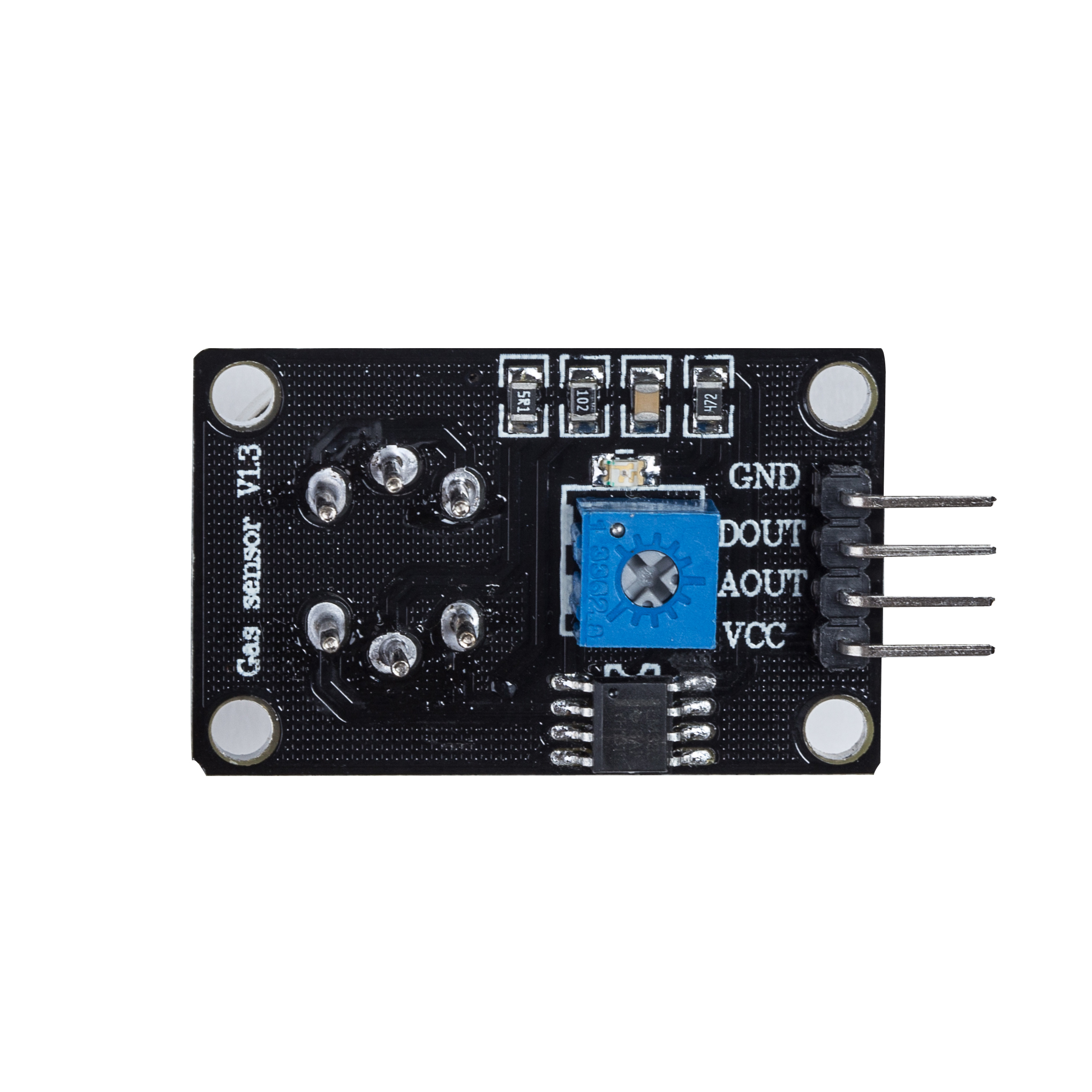• yes, R2 is 1k. Change RL_VALUE from 5 to 1.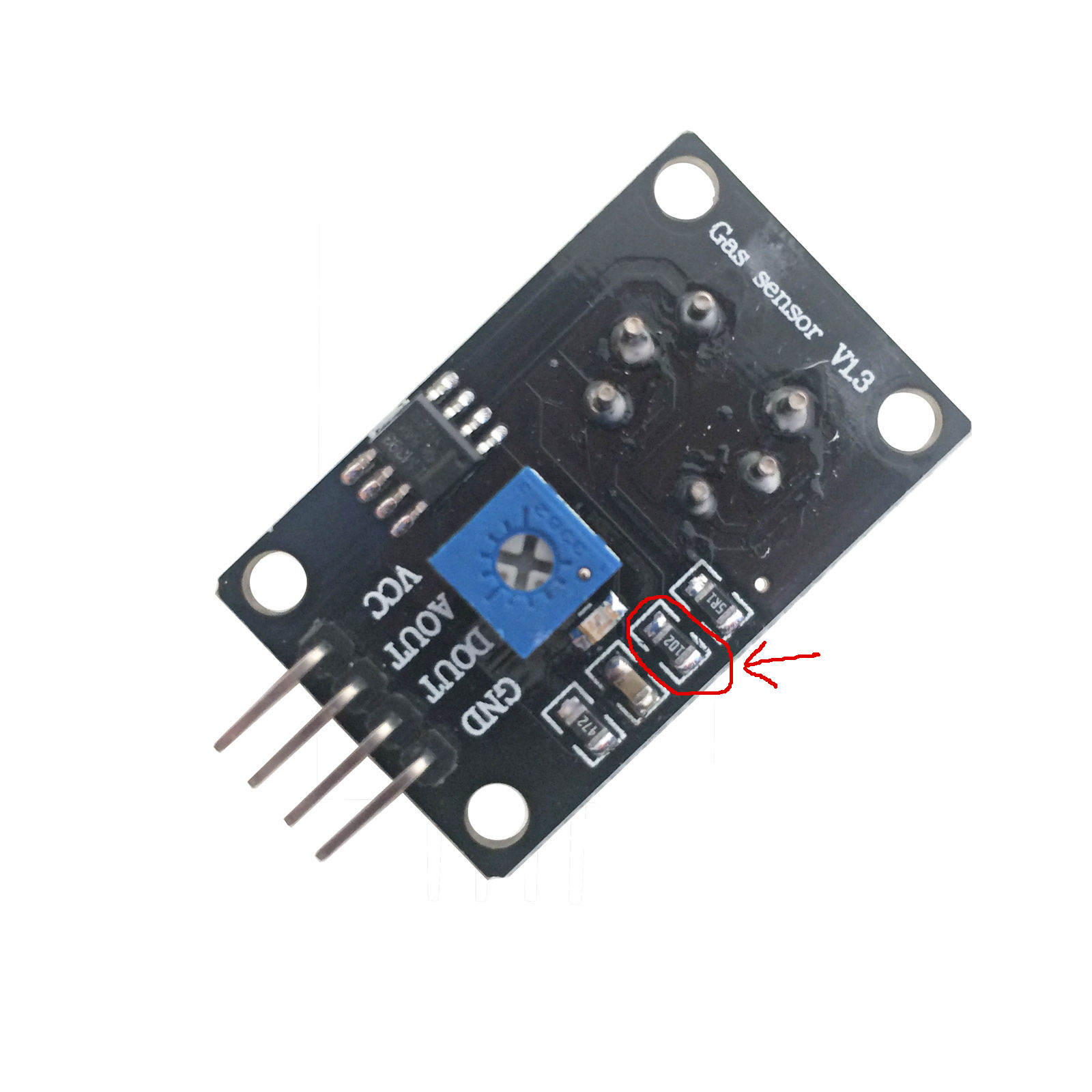I suggest you use the MQ-2 sensor library https://github.com/xerlay11/MQ-2-sensor-library and obviously change log and RL_VALUE.
Regards

• @zampedro
thank you and i am sorry for questions.
so in sketch this is enough just i change RL_VALUE 5 to 1 and change log to log10 ?
thank you for help

• It should be enough to read ppm from the sensor.

• @zampedro
thank you my friend . after 1 day this is work well.so i will test in next days. thank you

• @zampedro
hi friend after some day i have problem again with gas sensors mq2.
i use a air bag (full of gas) and put gas sensor in bag. some minutes i have 0ppm but after some minutes this is several report :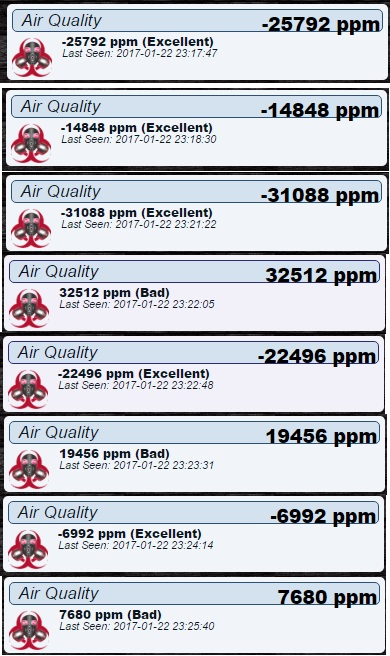i change RL_VALUE to 1 and use log10 in sketch but....

• i test again today. with a bag full of gas. but today gas sensor is 0 ppm and dont changewhat is problem ! thank you

• @Reza
You are sending a 16 bits signed integer, so the maximum value is 32767.

``````send(msg.set((int16_t)ceil(valMQ)));
``````

Try to send GAS_SMOKE instead of GAS_CO.
Pay attention to the power supply, the sensor need 5v 200mA ( i misured 130ma).

• @zampedro
so when must of 32767 so show me a - value ? but this is my problem yesterday.now i test again with a bag but dont any change and this is 0 ppm always . i dont use 5v pin in arduino for sensor. i use 5v from vcc power supply for vcc sensor .and power supply is 1.5A
for change to gas smoke this is enough change this line :

``````uint16_t valMQ = MQGetGasPercentage(MQRead(MQ_SENSOR_ANALOG_PIN) / Ro, GAS_CO);
``````

to

``````uint16_t valMQ = MQGetGasPercentage(MQRead(MQ_SENSOR_ANALOG_PIN) / Ro, GAS_SMOKE);
``````

?

• @Reza
you can't convert 16 bits unsigned integers greater than 32767 into 16 bits signed integers because they're treated as negative numbers according to 2's complement math.
You must check with a multimeter VCC(for supply voltage sanity) and DOUT( for ppm reading) on the sensor board.

I see two lines to be modified:

``````if (b == 0) {
uint16_t valMQ = MQGetGasPercentage(MQRead(MQ_SENSOR_ANALOG_PIN) / Ro, GAS_CO);

if (c == 0) {
uint16_t valMQ = MQGetGasPercentage(MQRead(MQ_SENSOR_ANALOG_PIN) / Ro, GAS_CO);
``````

• @zampedro
i use AOUT in wiring !in the site and sketch told use AOUT.... do you sure i must use DOUT?

• @Reza
sorry, I made a mistake. You have to check AOUT.

3

3

12

11

1

11

7